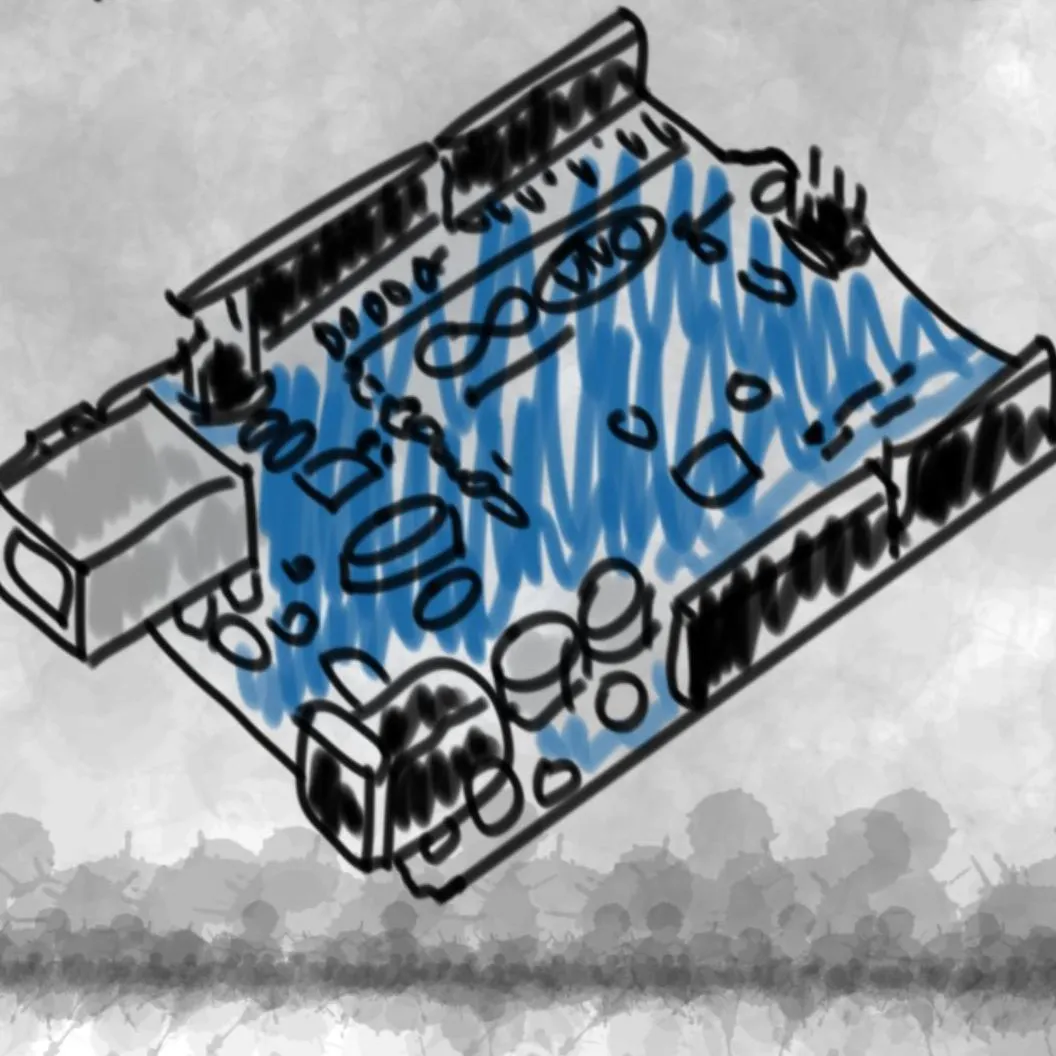# Arduino Paper Controller (Buttons + Slider)

This project allows you to draw your own controller to be used with anything. It utilizes capacitive sensing and graphite's conductivity.

IntermediateProtip1 hour16,726

## Things used in this project

### Hardware componentsArduino UNO
×1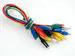Alligator Clips
×1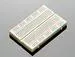×1
 Male/Male Jumper Wires
×1
 Resistor 10M Ohm
×3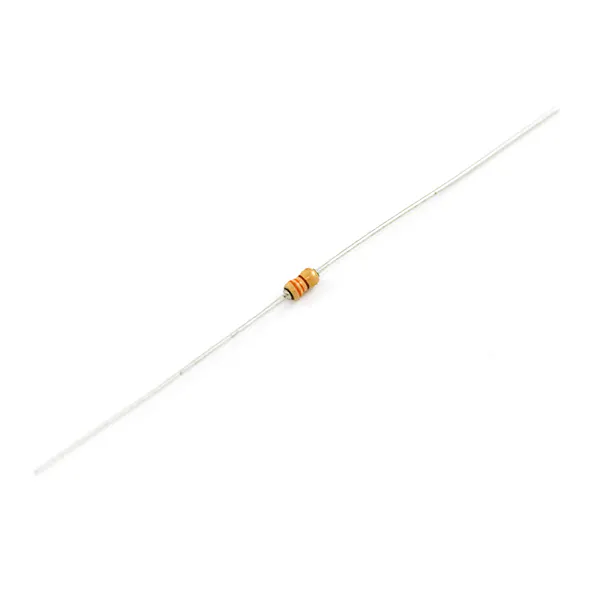Resistor 330 ohm
×2

### Software apps and online servicesArduino IDE

## Schematics

### Schematic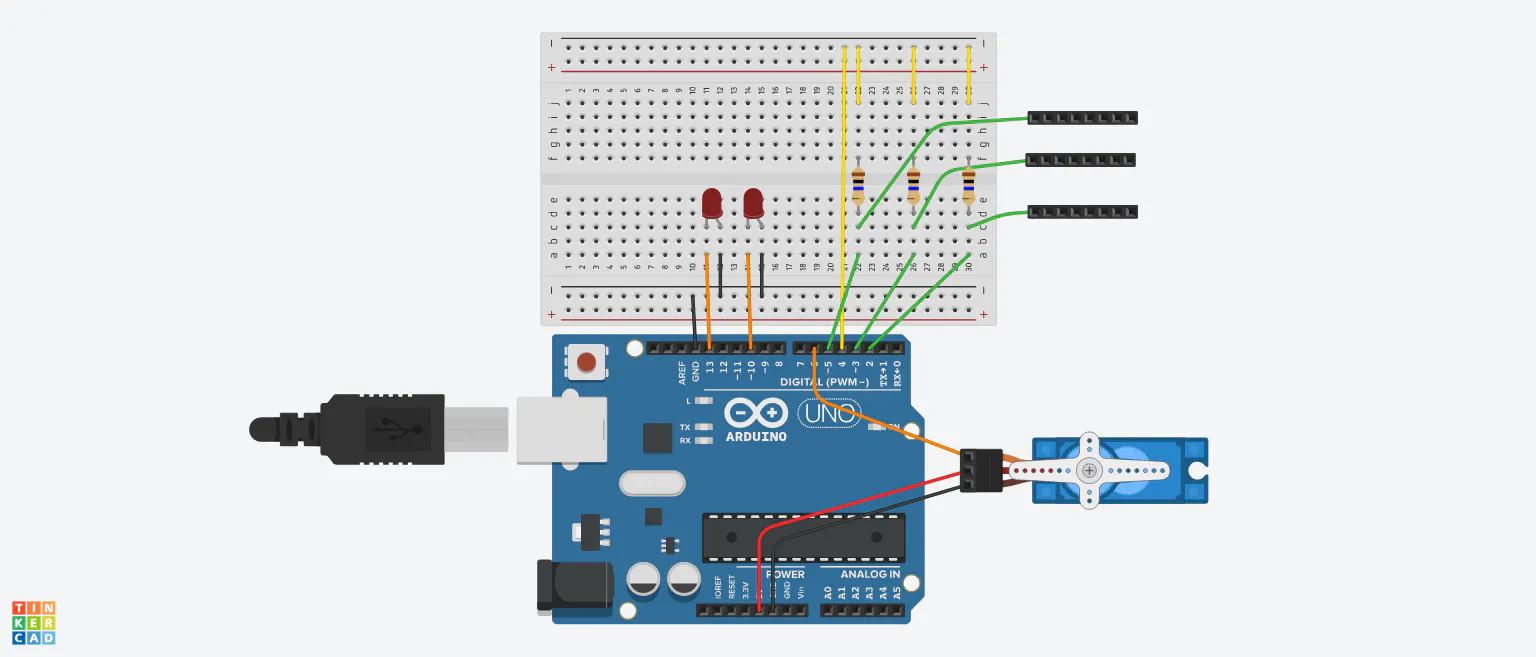## Code

### Code

Arduino
```#include <Servo.h>
#include <CapacitiveSensor.h>

Servo myservo;

CapacitiveSensor   button1 = CapacitiveSensor(4, 2);
CapacitiveSensor   button2 = CapacitiveSensor(4, 3);
CapacitiveSensor   slider = CapacitiveSensor(4, 5);

int total1val = 1000;//you'll need to edit these
int total2val = 1000;
int total3val1 = 100;
int total3val2 = 1000;

void setup() {
//button1.set_CS_AutocaL_Millis(0xFFFFFFFF);
Serial.begin(9600);
pinMode(10, OUTPUT);
pinMode(13, OUTPUT);
myservo.attach(6);
}

void loop() {
long start = millis();
long total1 =  button1.capacitiveSensor(1000);
long total2 =  button2.capacitiveSensor(1000);
long total = 0;
long total3 = 0;

for (int i = 1; i <= 10; i++) {//averages the value for the slide to make the servo smoother
total3 =  slider.capacitiveSensor(10000);
total = total + total3;
delay(1);
}

long avg = total / 10;
int angle;

Serial.print(millis() - start);
Serial.print("\t");

Serial.print(avg);
Serial.print("\t");
Serial.print(total2);
Serial.print("\t");
Serial.println(total3);

if (total1 > total1val) {
digitalWrite(13, HIGH);
}
else {
digitalWrite(13, LOW);
}

if (total2 > total2val) {
digitalWrite(10, HIGH);
}
else {
digitalWrite(10, LOW);
}

angle = map(avg, total3val1, total3val2, 180, 0);
myservo.write(angle);

delay(10);
}
```

## Credits

### millerman4487

10 projects • 73 followers